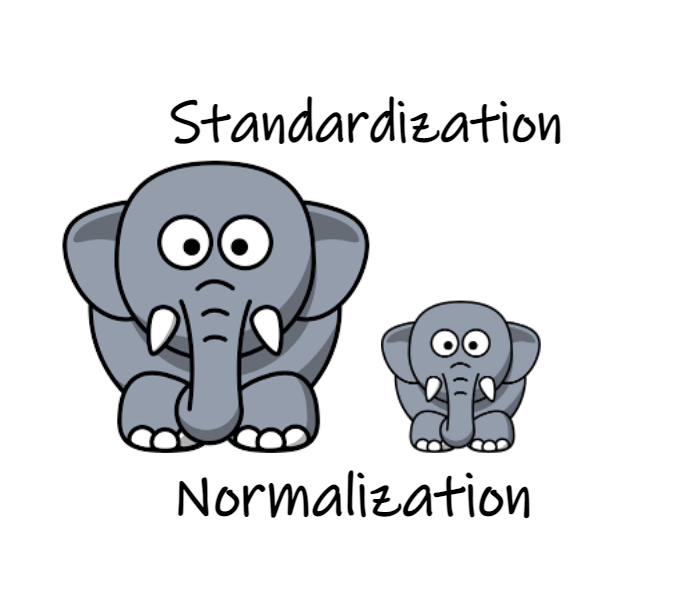Join thousands of AI enthusiasts and experts at the Learn AI Community.

## PublicationLatest

# Which Feature Scaling Technique To Use- Standardization vs Normalization

Last Updated on July 5, 2022 by Editorial Team

#### Author(s): Gowtham S R

Originally published on Towards AI the World’s Leading AI and Technology News and Media Company. If you are building an AI-related product or service, we invite you to consider becoming an AI sponsor. At Towards AI, we help scale AI and technology startups. Let us help you unleash your technology to the masses.

#### Is feature scaling mandatory?when to use standardization?when to use normalization? what will happen to the distribution of the data? what is the effect on outliers? Will the accuracy of the model increase?

When we begin the data science journey, we get a few questions regarding feature scaling which are really confusing.

The above questions are frequently asked in interviews too,, I will try to answer the above questions in this blog by providing suitable examples. We will use sklearn’s StandardScaler and MinMaxScaler.

Let us consider a dataset in which Age and Estimated Salary are the input features and we have to predict if the product is Purchased(output label) or not purchased.

Take a look at the first 5 rows of our data.

### What is StandardScaler?

StandardScaler or Z-Score Normalization is one of the feature scaling techniques, here the transformation of features is done by subtracting from the mean and dividing by standard deviation. This is often called Z-score normalization. The resulting data will have the mean as 0 and the standard deviation as 1.

So now we have seen the formula of standard scaling, Now we shall look at how it can be applied to our dataset.

First, we shall divide our data into train and test sets and apply a standard scaler.

Description of the dataset :

Note that the described method applied to X_train_scaled data shows that the mean is 0 and the standard deviation is 1 after applying the standard scaler.

Effect on the distribution of data:

From the above scatter plots and KDE plots we can note that the distribution of the data remains the same even after applying standard Scaler, only the scale changes.

How do different Machine Learning Models Perform before and After scaling?

In the above examples, the accuracy of Logistic regression and KNN increased significantly after scaling. But there was no effect on accuracy when the decision tree or random forest was used.

Effect on Outliers

The above plots show that the outliers in our data will be still the outliers even after applying the standard scaling. So, as data scientists, it is our responsibility to handle the outliers.

### What is MinMaxScaler?

MinMaxScaling(commonly used normalization technique) is one of the feature scaling techniques, it transforms features by subtracting from the minimum value of the data and dividing by (maximum minus minimum).

So now we have seen the formula min maxscaling, Now we shall look at how it can be applied to our dataset.

Description of the dataset :

Note that the minimum value of both the input features Age and Estimated Salary has become 0 and maximum value has become 1 after applying MinMax scaling.

Effect on Distribution of the data :

From the above scatter plots and KDE plots we can note that the distribution of the data remains the same even after applying minmax scaler, only the scale changes.

Effect of MinMaxScaler on different Machine Learning algorithms:

In the above examples, the accuracy of Logistic regression and KNN increased significantly after scaling. But there was no effect on accuracy when the decision tree or random forest was used.

Effect on outliers :

As shown above, there will not be any effect on outliers even after applying minmax scaling.

### Observations:

• The resulting data after standardization will have the mean 0 and a standard deviation of 1, whereas the resulting data after min-max scaling will have minimum value as0 and maximum value as 1 (Here the mean and standard deviation can be anything).
• The scatter plots and distplots above show that there will be no change in the distribution of data before and after applying the standard scaler or minmax scaler, only the scale changes.
• The feature scaling step has to be performed while applying algorithms where distance gets calculated (Eg: KNN, KMEANS), and involves gradient descent (Eg: Linear and Logistic regressions, neural networks).
• There will not be any effect of scaling when we use tree-based algorithms like decision trees or random forests.
• In the above examples, the accuracy of Logistic regression and KNN increased significantly after scaling. But there was no effect on accuracy when the decision tree or random forest was used.
• Outliers in the dataset will still remain an outlier even after applying the feature scaling methods, as data scientists, it is our responsibility to handle the outliers.
• There is no hard rule to tell which technique to use, but we need to check both standardization and normalization and decide based on the result which one to use.

If you have any confusion about the confusion matrix, precision, and recall, then please read the below blog.

Confusion Matrix

If you want to know how to analyze the IPL data, please read the below blog.

Virat Kohli vs KL Rahul

Get the data science book click here

Mlearning.ai Submission SuggestionsWhich Feature Scaling Technique To Use- Standardization vs Normalization was originally published in Towards AI on Medium, where people are continuing the conversation by highlighting and responding to this story.

Join thousands of data leaders on the AI newsletter. It’s free, we don’t spam, and we never share your email address. Keep up to date with the latest work in AI. From research to projects and ideas. If you are building an AI startup, an AI-related product, or a service, we invite you to consider becoming a sponsor.

Published via Towards AI